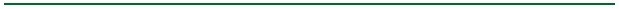Fundamentals of Queueing Theory Fourth Edition Donald Gross John F. Shortle James M. Thompson Carl M. Harris (c) 2008 by John Wiley & Sons, Inc, Hoboken, NJ.• Chapter 1: Introduction
• Chapter 2: Simple Markovian Queueing Models
• Chapter 3: Advanced Markovian Queueing Models
• Chapter 4: Networks, Series, and Cyclic Queues
• Chapter 5: General Arrival or Service Patterns
• Chapter 6: General Models and Theoretical Topics
• Chapter 7: Bounds and Approximations
• Chapter 8: Numerical Techniques and Simulation

### Key Changes From 3rd Edition

• Chapter 2 (Simple Markovian Queueing Models)
• New section on choosing the number of servers (or the square-root law)
• New sub-section on computational issues of the Erlang-B and Erlang-C formulas
• Section on birth-death models moved from Chapter 1 to Chapter 2
• Chapter 3 (Advanced Markovian Queueing Models)
• New section on retrial queues
• Section on priority queues substantially rewritten; several other sections rewritten for clarity
• Chapter 5 (General Arrival or Service Patterns)
• Section on M/G/1 queue rewritten for clarity, including new alternate derivation of PK formula
• New discussion of level-crossing methods
• Chapter 7 (Bounds and Approximations)
• The former Chapter 7 has been split into two chapters, 7 and 8.
• New section on network approximations
• Chapter 8 (Numerical Techniques and Simulation)
• New section on numerical inversion of transforms
• Many new problems throughout text
• Many added figures and editing for clarity throughout text

### Errata: First Printing

• Index references are off by several pages in many cases. See corrected index (sorry!).
• p 251. Equation at the bottom of the page is missing 'dy'
• p. 254. Statement that “R(y) is called the staying function" should read "1 - R(y) is called the staying function" to be consistent with Brill (2008). However, the discussion is internally consistent.
• p. 234 and 245. “Theorem 1.2 of Section 1.10” should be “Theorem 1.2 of Section 1.9.6.”
• p. ix. According to the table of contents, the index is on p. 495. It is really on p. 493.

### Errata: First and Other Printings

• p. 146, two lines before equation 3.36. "W" and "L" are reversed in the Little's-law relations. They should read:
• Correct: "Lq(i) = &lambdai Wq(i)" and "L(i) = &lambdai W(i)"
• Incorrect: "Wq(i) = &lambdai Lq(i)" and "W(i) = &lambdai L(i)"
• p. 127, last paragraph. The paragraph starts "Prior to showing an example, ...". This phrase should be omitted. The example was given previously (Example 3.4).
• p. 81, Example 2.6. The equation for L should read: "L = ... = 6918/1141 = 6.06 cars" (i.e., the fraction should have 6918 in the numerator, not 9606). The final numerical values for W and the balking rate are also off in the last digit. They should read: W = 12.2 min (instead of 12.3) and balking rate = 30.3 cars/h (instead of 30.4 cars/h). Finally, in the last equation, pk should be pK.
• p. 196-197. In equation 4.17 and 4.18 and Example 4.4, &rhoi should be replaced by ri, where ri is interpreted as the offered load (ri = &lambdai / &mui) and &rhoi is interpreted as the utilization (&rhoi = &lambdai / ci &mui). The example is worked correctly, but the notation is sloppy in the sense that &rhoi should represent the offered load in 4.17 and 4.18 and Example 4.4, not the utilization.
• p. 136, Example 3.8. This example is technically correct. But it may make more sense if the line before the equation reads "...and is given by (3.22)" (not 3.23). The equation as it is written is obtained by solving (3.23) for Wq which puts a &lambda in the denominator. But it's probably clearer to obtain the same result (Wq = 2 hours) from (3.22). In the written equation, this involves replacing 16/25 with 4/5 (rho) in the numerator and replacing 6/5 with 3/2 (mu) in the denominator.